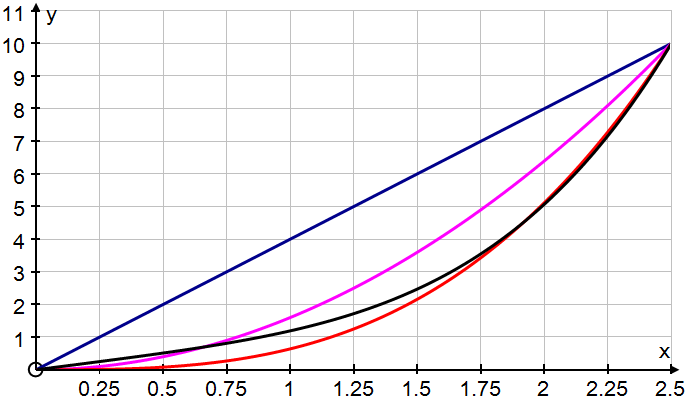#### You may also like### Brimful 2

Which of these infinitely deep vessels will eventually full up?

# Brimful

##### Age 16 to 18Challenge Level

Constants $A, B, C, D$ are chosen so that the following $4$ curves pass through the point $(2.5, 10)$ $$y = Ax\quad y = Bx^2 \quad y = Cx^3\quad y = Dx^4+x$$
What values must the constants take? Can you identify each curve in the following accurately drawn chart?These curves are now used to design some mathematical vessels of height $10$ by rotating the curves about the $y$ axis. Assuming that $x$ and $y$ are measured in centimetres, what are the volumes of the vessels?

Water is poured slowly at a rate of 1cm$^3$ per minute into these vessels. At what depth of water, to the nearest mm, will each of them be half full? Do your results make sense from the diagram?